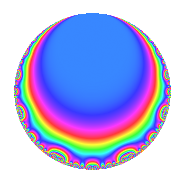Properties

 Label 6040.2.a.kLevel 6040 Weight 2 Character orbit 6040.a Self dual Yes Analytic conductor 48.230 Analytic rank 1 Dimension 2 CM No Inner twists 1

Related objects

Newspace parameters

 Level: $$N$$ = $$6040 = 2^{3} \cdot 5 \cdot 151$$ Weight: $$k$$ = $$2$$ Character orbit: $$[\chi]$$ = 6040.a (trivial)

Newform invariants

 Self dual: Yes Analytic conductor: $$48.2296428209$$ Analytic rank: $$1$$ Dimension: $$2$$ Coefficient field: $$\Q(\sqrt{17})$$ Coefficient ring: $$\Z[a_1, a_2, a_3]$$ Coefficient ring index: $$1$$ Fricke sign: $$1$$ Sato-Tate group: $\mathrm{SU}(2)$

$q$-expansion

Coefficients of the $$q$$-expansion are expressed in terms of $$\beta = \frac{1}{2}(1 + \sqrt{17})$$. We also show the integral $$q$$-expansion of the trace form.

 $$f(q)$$ $$=$$ $$q$$ $$-\beta q^{3}$$ $$+ q^{5}$$ $$+ ( -1 + \beta ) q^{7}$$ $$+ ( 1 + \beta ) q^{9}$$ $$+O(q^{10})$$ $$q$$ $$-\beta q^{3}$$ $$+ q^{5}$$ $$+ ( -1 + \beta ) q^{7}$$ $$+ ( 1 + \beta ) q^{9}$$ $$+ q^{11}$$ $$- q^{13}$$ $$-\beta q^{15}$$ $$-2 q^{17}$$ $$+ ( 4 - \beta ) q^{19}$$ $$-4 q^{21}$$ $$+ ( -1 + 2 \beta ) q^{23}$$ $$+ q^{25}$$ $$+ ( -4 + \beta ) q^{27}$$ $$-3 q^{29}$$ $$+ ( -1 - 2 \beta ) q^{31}$$ $$-\beta q^{33}$$ $$+ ( -1 + \beta ) q^{35}$$ $$+ ( 2 - 4 \beta ) q^{37}$$ $$+ \beta q^{39}$$ $$+ ( -6 + 2 \beta ) q^{41}$$ $$-2 q^{43}$$ $$+ ( 1 + \beta ) q^{45}$$ $$+ ( -4 + 2 \beta ) q^{47}$$ $$+ ( -2 - \beta ) q^{49}$$ $$+ 2 \beta q^{51}$$ $$+ 2 q^{53}$$ $$+ q^{55}$$ $$+ ( 4 - 3 \beta ) q^{57}$$ $$-9 q^{59}$$ $$+ ( 4 + 2 \beta ) q^{61}$$ $$+ ( 3 + \beta ) q^{63}$$ $$- q^{65}$$ $$+ ( -1 + 4 \beta ) q^{67}$$ $$+ ( -8 - \beta ) q^{69}$$ $$-2 \beta q^{71}$$ $$+ ( 3 - 4 \beta ) q^{73}$$ $$-\beta q^{75}$$ $$+ ( -1 + \beta ) q^{77}$$ $$-8 q^{79}$$ $$-7 q^{81}$$ $$+ ( 3 + 4 \beta ) q^{83}$$ $$-2 q^{85}$$ $$+ 3 \beta q^{87}$$ $$+ ( -10 - 2 \beta ) q^{89}$$ $$+ ( 1 - \beta ) q^{91}$$ $$+ ( 8 + 3 \beta ) q^{93}$$ $$+ ( 4 - \beta ) q^{95}$$ $$+ ( -4 + 4 \beta ) q^{97}$$ $$+ ( 1 + \beta ) q^{99}$$ $$+O(q^{100})$$ $$\operatorname{Tr}(f)(q)$$ $$=$$ $$2q$$ $$\mathstrut -\mathstrut q^{3}$$ $$\mathstrut +\mathstrut 2q^{5}$$ $$\mathstrut -\mathstrut q^{7}$$ $$\mathstrut +\mathstrut 3q^{9}$$ $$\mathstrut +\mathstrut O(q^{10})$$ $$2q$$ $$\mathstrut -\mathstrut q^{3}$$ $$\mathstrut +\mathstrut 2q^{5}$$ $$\mathstrut -\mathstrut q^{7}$$ $$\mathstrut +\mathstrut 3q^{9}$$ $$\mathstrut +\mathstrut 2q^{11}$$ $$\mathstrut -\mathstrut 2q^{13}$$ $$\mathstrut -\mathstrut q^{15}$$ $$\mathstrut -\mathstrut 4q^{17}$$ $$\mathstrut +\mathstrut 7q^{19}$$ $$\mathstrut -\mathstrut 8q^{21}$$ $$\mathstrut +\mathstrut 2q^{25}$$ $$\mathstrut -\mathstrut 7q^{27}$$ $$\mathstrut -\mathstrut 6q^{29}$$ $$\mathstrut -\mathstrut 4q^{31}$$ $$\mathstrut -\mathstrut q^{33}$$ $$\mathstrut -\mathstrut q^{35}$$ $$\mathstrut +\mathstrut q^{39}$$ $$\mathstrut -\mathstrut 10q^{41}$$ $$\mathstrut -\mathstrut 4q^{43}$$ $$\mathstrut +\mathstrut 3q^{45}$$ $$\mathstrut -\mathstrut 6q^{47}$$ $$\mathstrut -\mathstrut 5q^{49}$$ $$\mathstrut +\mathstrut 2q^{51}$$ $$\mathstrut +\mathstrut 4q^{53}$$ $$\mathstrut +\mathstrut 2q^{55}$$ $$\mathstrut +\mathstrut 5q^{57}$$ $$\mathstrut -\mathstrut 18q^{59}$$ $$\mathstrut +\mathstrut 10q^{61}$$ $$\mathstrut +\mathstrut 7q^{63}$$ $$\mathstrut -\mathstrut 2q^{65}$$ $$\mathstrut +\mathstrut 2q^{67}$$ $$\mathstrut -\mathstrut 17q^{69}$$ $$\mathstrut -\mathstrut 2q^{71}$$ $$\mathstrut +\mathstrut 2q^{73}$$ $$\mathstrut -\mathstrut q^{75}$$ $$\mathstrut -\mathstrut q^{77}$$ $$\mathstrut -\mathstrut 16q^{79}$$ $$\mathstrut -\mathstrut 14q^{81}$$ $$\mathstrut +\mathstrut 10q^{83}$$ $$\mathstrut -\mathstrut 4q^{85}$$ $$\mathstrut +\mathstrut 3q^{87}$$ $$\mathstrut -\mathstrut 22q^{89}$$ $$\mathstrut +\mathstrut q^{91}$$ $$\mathstrut +\mathstrut 19q^{93}$$ $$\mathstrut +\mathstrut 7q^{95}$$ $$\mathstrut -\mathstrut 4q^{97}$$ $$\mathstrut +\mathstrut 3q^{99}$$ $$\mathstrut +\mathstrut O(q^{100})$$

Embeddings

For each embedding $$\iota_m$$ of the coefficient field, the values $$\iota_m(a_n)$$ are shown below.

For more information on an embedded modular form you can click on its label.

Label $$\iota_m(\nu)$$ $$a_{2}$$ $$a_{3}$$ $$a_{4}$$ $$a_{5}$$ $$a_{6}$$ $$a_{7}$$ $$a_{8}$$ $$a_{9}$$ $$a_{10}$$
1.1
 2.56155 −1.56155
0 −2.56155 0 1.00000 0 1.56155 0 3.56155 0
1.2 0 1.56155 0 1.00000 0 −2.56155 0 −0.561553 0
 $$n$$: e.g. 2-40 or 990-1000 Significant digits: Format: Complex embeddings Normalized embeddings Satake parameters Satake angles

Inner twists

This newform does not admit any (nontrivial) inner twists.

Atkin-Lehner signs

$$p$$ Sign
$$2$$ $$-1$$
$$5$$ $$-1$$
$$151$$ $$1$$

Hecke kernels

This newform can be constructed as the intersection of the kernels of the following linear operators acting on $$S_{2}^{\mathrm{new}}(\Gamma_0(6040))$$:

 $$T_{3}^{2}$$ $$\mathstrut +\mathstrut T_{3}$$ $$\mathstrut -\mathstrut 4$$ $$T_{7}^{2}$$ $$\mathstrut +\mathstrut T_{7}$$ $$\mathstrut -\mathstrut 4$$ $$T_{11}$$ $$\mathstrut -\mathstrut 1$$